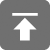位数组(bit array)是一种常用的数据结构，逻辑上可以理解为一个元素取值只可能为0和1的数组。在一些内存紧张的场景下是一个不错的选择。

comp.lang.c上介绍了一个位数组的简单实现

#include <limits.h>
//  If you don't have <limits.h>, try using 8 for CHAR_BIT.

#define BITMASK(b) (1 << ((b) % CHAR_BIT))
#define BITSLOT(b) ((b) / CHAR_BIT)
#define BITSET(a, b) ((a)[BITSLOT(b)] |= BITMASK(b))
#define BITCLEAR(a, b) ((a)[BITSLOT(b)] &= ~BITMASK(b))
#define BITTEST(a, b) ((a)[BITSLOT(b)] & BITMASK(b))
#define BITNSLOTS(nb) ((nb + CHAR_BIT - 1) / CHAR_BIT)

其中头文件limits.h中定义了一些C语言常用的极限值，例如各种整数类型的最大值和最小值，一个char型占用的bit数，等。

以上几个宏的实现，都不难理解。注意最后一个宏BITNSLOTS(nb)的实现，这种用法在一些图形图像处理或存储中比较常见；因为这里存储bit array的大小只可 能是8的倍数，而图形图像处理中，为了保证访问内存的速度，常常将数据进行$2^n$字节对齐，用法和此处有些类似。

下面来看一个使用的例子，用埃拉托斯特尼筛法求素数：

#include <stdio.h>
#include <string.h>

#define MAX 10000

int main()
{
//申请位数组
char bitarray[BITNSLOTS(MAX)];
int i, j;

memset(bitarray, 0, BITNSLOTS(MAX));

for(i = 2; i < MAX; i++)
{
if(!BITTEST(bitarray, i))
{
printf("%d\n", i);
for(j = i + i; j < MAX; j += i)
{
BITSET(bitarray, j);
}
}
}
return 0;
}

另外，还可以对两个位数组整体进行逻辑运算，例如：

for (int i = 0; i < BITNSLOT(ARRAY_SIZE); ++i)
{
arr_1[i] |= arr_2[i];
arr_2[i] &= arr_3[i];
}

### Post Info